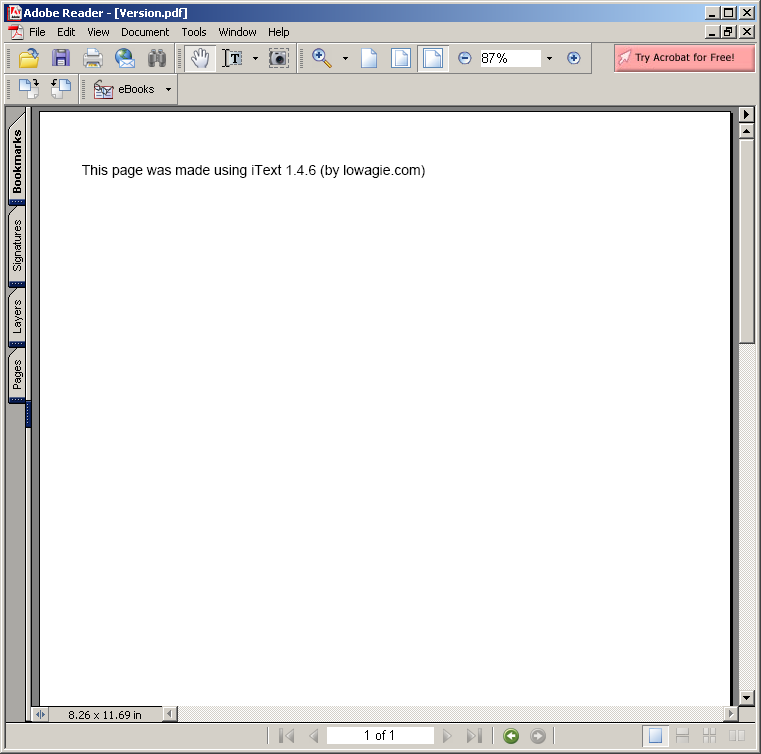# Sieve : Algorithms « Collections Data Structure « Java

Sieve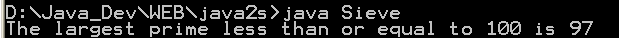```
/*
* This code is from the book Java Examples in a Nutshell, 2nd Edition.
* It is provided AS-IS, WITHOUT ANY WARRANTY either expressed or implied.
* You may study, use, and modify it for any non-commercial purpose.
* You may distribute it non-commercially as long as you retain this notice.
* For a commercial use license, or to purchase the book (recommended),
* visit http://www.davidflanagan.com/javaexamples2.
*/

/**
* This program computes prime numbers using the Sieve of Eratosthenes
* algorithm: rule out multiples of all lower prime numbers, and anything
* remaining is a prime. It prints out the largest prime number less than or
* equal to the supplied command-line argument.
*/
public class Sieve {
public static void main(String[] args) {
// We will compute all primes less than the value specified on the
// command line, or, if no argument, all primes less than 100.
int max = 100; // Assign a default value
try {
max = Integer.parseInt(args);
} // Parse user-supplied arg
catch (Exception e) {
} // Silently ignore exceptions.

// Create an array that specifies whether each number is prime or not.
boolean[] isprime = new boolean[max + 1];

// Assume that all numbers are primes, until proven otherwise.
for (int i = 0; i <= max; i++)
isprime[i] = true;

// However, we know that 0 and 1 are not primes. Make a note of it.
isprime = isprime = false;

// To compute all primes less than max, we need to rule out
// multiples of all integers less than the square root of max.
int n = (int) Math.ceil(Math.sqrt(max)); // See java.lang.Math class

// Now, for each integer i from 0 to n:
//   If i is a prime, then none of its multiples are primes,
//   so indicate this in the array. If i is not a prime, then
//   its multiples have already been ruled out by one of the
//   prime factors of i, so we can skip this case.
for (int i = 0; i <= n; i++) {
if (isprime[i]) // If i is a prime,
for (int j = 2 * i; j <= max; j = j + i)
// loop through multiples
isprime[j] = false; // they are not prime.
}

// Now go look for the largest prime:
int largest;
for (largest = max; !isprime[largest]; largest--)
; // empty loop body

// Output the result
System.out.println("The largest prime less than or equal to " + max
+ " is " + largest);
}
}

```

### Related examples in the same category

 1 Anagrams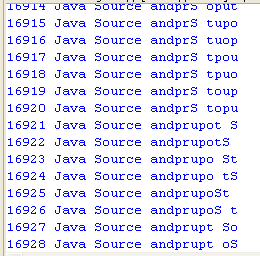2 Hanoi puzzle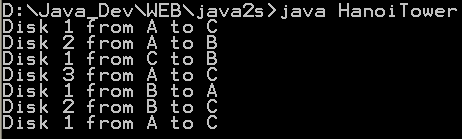3 Fibonacci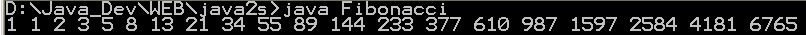4 Find connections using a depth-first search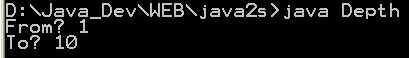5 Find connections using hill climbing. 6 Find optimal solution using least-cost 7 Find the lost keys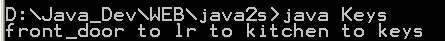8 Compute the area of a triangle using Heron's Formula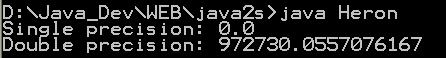9 Compute prime numbers 10 Print a table of fahrenheit and celsius temperatures 1 11 Print a table of fahrenheit and celsius temperatures 2 12 Print a table of Fahrenheit and Celsius temperatures 3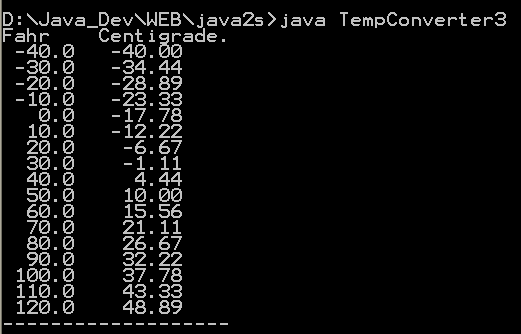13 Soundex - the Soundex Algorithm, as described by Knuth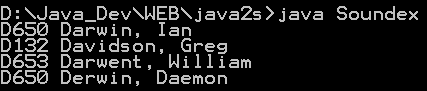14 A programmable Finite State Machine implementation. 15 An extendable Graph datastructure. 16 Utilities for flop (floating-point operation) counting. 17 LU Decomposition 18 Reverse Polish Notation 19 Permutator test 20 implements the LZF lossless data compression algorithm 21 Linear Interpolation 22 Utility class for generating the k-subsets of the numbers 0 to n 23 Version